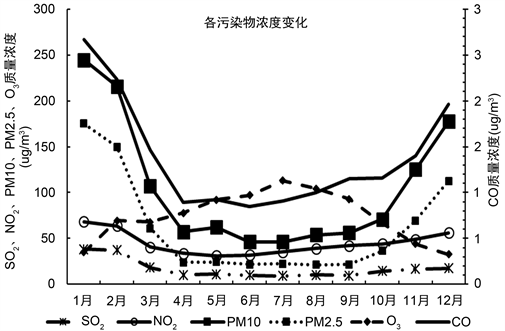﻿ 2016~2018年昌吉市空气污染预报及效果检验

# 2016~2018年昌吉市空气污染预报及效果检验The Forecast and Effect Text of Air Pollution in Changji from 2016 to 2018 Years

Abstract: Based on the correlation analysis between daily air quality concentration and corresponding period of the meteorological factors at high altitude and ground in Changji City from January 2016 to December 2018, the multiple linear regression equation was established by SPSS software, and the prediction effect was tested. The concrete conclusions were as follows: 1) The change of con-centration of various pollutants was basically V-shaped, and winter was the season in which the mass concentration of pollutants increased sharply; the change trend of ozone was “inverted V” type, and summer was the season with the highest mass concentration; the mass concentration of PM10 and PM2.5 increased obviously in winter; 2) PM10 as the primary pollutant appeared most frequently in spring, while the ozone as the primary pollutant appeared most frequent pollutant in summer, PM10 as the primary pollutant was appeared in autumn again, the most high frequency of PM2.5 was in winter, and SO2 was not as a primary pollutant in four seasons; 3) The air quality in spring, summer and autumn was mainly secondary; 4) According to the test of forecast effect, the forecast value was higher, the forecast error of PM10 concentration in summer was bigger, and the other pollutants were slightly higher; the forecast result was slightly higher than the actual situation, the forecast effect of summer half year was better than winter half year, the forecast effect of PM10, PM2.5 was worse, the forecast effect of SO2, CO and NO2 was better; the prediction effect for air quality with serious pollution was poor.

1. 引言

2. 资料及方法说明

2.1. 污染物质量浓度资料

2.2. 气象因子的选取

2.3. 研究的方法

${T}_{ME}=\frac{1}{N}\underset{i=1}{\overset{N}{\sum }}\left({F}_{i}-{O}_{i}\right)$ (1)

${T}_{RMSE}=\sqrt{\frac{1}{N}\underset{i=1}{\overset{N}{\sum }}{\left({F}_{i}-{O}_{i}\right)}^{2}}$ (2)

3. 空气质量变化特征Figure 1. Characteristics of mass concentration changes of six types of pollutantsTable 1. The main pollutants and air quality frequency of various grades in Changji City from 2016 to 2017

4. 逐步回归方法制作空气质量预报

4.1. 空气质量预报

$\begin{array}{l}{\text{SO}}_{2}=-51.887+1052\ast {x}_{1}-0.074\ast {x}_{2}-2.623\ast {x}_{3}+0.569\ast {x}_{4}-4.611\ast {x}_{5}+1.485\ast {x}_{6}-4.402\ast {x}_{7}\\ \text{}+0.6112\ast {x}_{8}+3.313\ast {x}_{9}+0.112\ast {x}_{10}+0.020\ast {x}_{11}-0.339\ast {x}_{12}+3.158\ast {x}_{13}\end{array}$ (3)

${\text{NO}}_{2}=57.064-1.307\ast {x}_{14}+1.946\ast {x}_{1}+0.225\ast {x}_{15}-5.994\ast {x}_{16}+0.851\ast {x}_{17}+0.025\ast {x}_{18}-0.048\ast {x}_{2}$ (4)

$\begin{array}{l}\text{PM10}=460.881-52.958\ast {x}_{16}+5.668\ast {x}_{18}-18.421\ast {x}_{20}-0.276\ast {x}_{2}\\ \text{}+3.775\ast {x}_{21}-7.013\ast {x}_{14}+4.911105384750788\ast {x}_{22}\end{array}$ (5)

$\text{PM2}\text{.5}=280.041-5.009\ast {x}_{14}+3.698\ast {x}_{19}-0.219\ast {x}_{23}-25.343\ast {x}_{16}+3.209\ast {x}_{21}-5.832\ast {x}_{24}$ (6)

${\text{O}}_{3}=419.570-26.654\ast {x}_{20}+2.235\ast {x}_{22}-10.807\ast {x}_{26}-3.832\ast {x}_{27}+16.154\ast {x}_{28}-0.500\ast {x}_{12}+6.869\ast {x}_{29}$ (7)

4.2. 预报结果分析

4.2.1. 平均误差与均方根误差Table 2. Forecast errors of six types of pollutants

4.2.2. AQI级别预报准确率Table 3. Forecast accuracy of air quality grade

5. 逐步回归法预报污染物质量浓度的误差分析

6. 结论

1) 从六类污染物质量浓度的月变化可以看出，各类污染物变化基本呈V型，冬季是各类污染物质量浓度剧增的季节；其中臭氧的变化趋势为倒V型，夏季是质量浓度最高的季节；CO、SO2的质量浓度较其他污染物浓度偏低；PM10、PM2.5的质量浓度在冬季增加明显，冬季为夏季的5至6倍；NO2质量浓度变化较其他类变化略微平缓，春季是浓度最小的季节。

2) PM10作为首要污染物在春季出现的次数最多，夏季中臭氧的作为首要污染物出现次数最多，为148次；秋季中PM10再次转为首要污染物；冬季PM2.5出现频次最多，为251次，占94%，几乎冬季首要污染物均为PM2.5。四季中SO2未作为首要污染物出现。

3) 从各等级空气质量出现频次上来看，春季、夏季和秋季空气质量主要以二级(良)为主；冬季空气质量为五级(重度污染)出现了99次，占总频次的37%，出现了18次严重污染。夏季空气质量最好，冬季受大气逆温层、少降水、多阴雾、小风等原因的影响，空气质量最差。

4) 通过检验预报方程的效果来看，预报值偏高，夏季PM10浓度预报值误差较大，其他类污染物为略偏高；预报结果较实况略偏高，夏半年的预报效果优于冬半年，PM10、PM2.5预报效果较差，SO2、CO和NO2的预报效果较好；针对严重污染的空气质量预报效果较差。

NOTES

*通讯作者。

 魏璐, 朱伟军, 陈海山. 郑州市空气质量统计预报方法探讨[J]. 南京气象学院学报, 2009, 32(2): 314-320.

 王芳, 程水源, 李明君, 等. 遗传算法优化神经网络用于大气污染预报[J]. 北京工业大学学报, 2009, 35(9): 1231-1234.

 杨民, 王庆梅, 马印飞. 兰州市大气污染气象潜势预报研究[J]. 甘肃环境研究与监测, 2002, 15(3): 155-157.

 黄晓娴, 王体健, 江飞. 空气污染潜势–统计结合预报模型的建立及应用[J]. 中国环境科学, 2012, 32(8): 1400-1408.

 程水源, 郝瑞霞, 杨智明. 关于大气污染预测方法的新探讨[J]. 环境科学丛刊, 1991, 12(2): 34-38.

 胡春梅, 刘德, 陈得劲. 重庆市空气污染扩散气象条件指标研究[J]. 气象科技, 2009, 37(6): 665-669.

 程水源, 郝瑞霞. 城市大气污染物长期平均浓度计算方法的研究[J]. 环境科学丛刊, 1990, 11(2): 44-48.

 王迎春, 孟燕军, 赵习方. 北京市空气污染业务预报方法[J]. 气象科技, 2001, 29(4): 42-46.

 刘实, 王宁, 朱其文, 等. 长春市空气污染潜势预报的统计模型研究[J]. 气象, 2002, 28(1): 8-12.

 李琼, 李福桥, 叶燕翔, 等. 珠江三角洲地区天气类型与污染潜势及污染浓度的关系[J]. 热带气象学报, 1999, 15(4): 363-369.

 徐大梅, 朱蓉. 大气平流扩散的箱格预报模型与污染潜势指数预报[J]. 应用气象学报, 2000, 11(1): 1-11.

 周小珊, 张立祥, 刘万军. 利用中尺度模型做辽宁省空气污染气象条件预报[J]. 南京气象学院学报, 2002, 25(1): 129-135.

 孙明华, 徐大海, 朱蓉, 等. 城市空气臭氧污染业务预报方案研究[J]. 气象, 2002, 28(4): 3-8.

 刘新春, 钟玉婷, 河清, 等. 乌鲁木齐大气总悬浮颗粒物(TSP)离子化学组分及影响因素[J]. 干旱区研究, 2012, 29(4): 713-720.

 阿依古丽•艾力亚斯, 玉米提•哈力克, 迈迪娜•吐尔逊. 阿克苏市空气污染及其变化分析[J]. 干旱区研究, 2016, 33(3): 649-654.

 魏疆, 陈学刚, 任泉, 等. 乌鲁木齐市能源结构调整对冬季大气污染物浓度的影响[J]. 干旱区研究, 2015, 32(1): 155-160.

 李帅, 郭志忠, 胡列群, 等. 乌鲁木齐冬季大气中黑炭气溶胶污染特征[J]. 干旱区研究, 2012, 29(4): 727-734.

 李四清, 张向东. 气象因子与空气质量监测预报的关系[J]. 现代农业科技, 2007(16): 233-236.

 魏疆, 王国华, 任泉, 等. 乌鲁木齐市大气污染物浓度计量模型研究[J]. 干旱区研究, 2011, 28(5): 896-900.

 赵克明, 李霞, 杨静. 乌鲁木齐大气最大混合层厚度变化的环境响应[J]. 干旱区研究, 2011, 28(3): 509-513.

 赵克明, 李霞, 孙鸣婧, 等. 乌鲁木齐冬季浅薄型焚风对大气扩散条件及空气质量的影响[J]. 沙漠与绿洲气象, 2019, 13(1): 13-20.

 巩庆, 范金霞, 林卫, 等. 2014-2015年新疆库尔勒市空气质量的时空分布特征[J]. 沙漠与绿洲气象, 2017, 11(5): 77-82.

 屠月青, 穆彩云. 哈密市空气污染物浓度分布特征及其与气象因子的关系[J]. 沙漠与绿洲气象, 2010, 4(6): 42-46.

 杨兴华, 河清, 刘涛, 等. 乌鲁木齐市冬季混合层厚度及对大气污染影响的个例分析[J]. 沙漠与绿洲气象, 2010, 4(4): 18-21.

 赵惠芳, 陈雅莲, 唐会荣, 等. 晋江城市空气质量污染潜势统计预报方法初探[J]. 气象与环境学报, 2009, 25(5): 27-30.

 张朝能, 胡兵. 空气质量预报方法综述[J]. 云南环境科学, 2003, 22(2): 55-57.

 许杨, 王凯, 贾桥莲, 等. 2007-2008年武汉市空气质量预报及检验[J]. 气象与环境学报, 2012, 28(2): 81-84.

Top